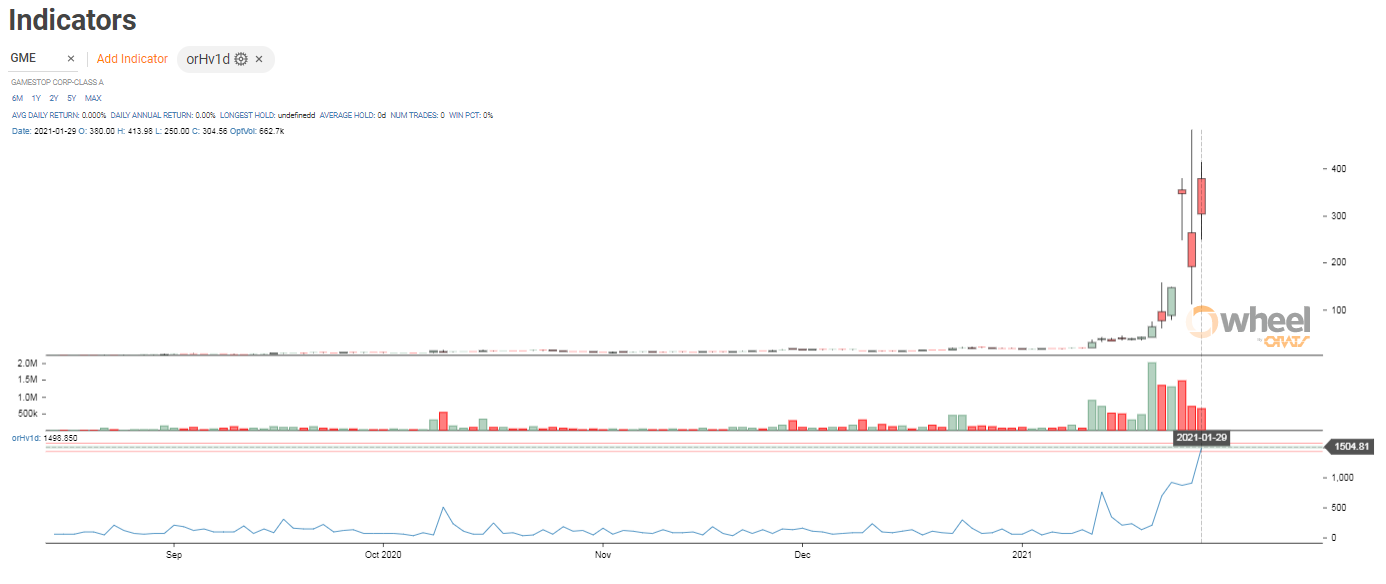GameStop (GME) was nuts yesterday. The major brokerages had severely limited retail’s ability to trade and the short hedge funds had the upper hand driving the stock down from near \$500 to \$150. Implied volatility in the options soared to levels I have never seen in my 30 year history of tracking options, and the actual volatility was like nothing I have ever seen either.

I never thought I would buy an option trading at a 1000% implied volatility but that is what I did, closing out my last position, a call spread, one option at a time.

Even though I had a relatively low risk position, I wanted to get out before expiration. Many unwitting options traders do not understand that even though their options expired and in a spread canceled the positions in the exercised stock, the long options holders can choose to exercise or not exercise an option thereby creating a stock position that was unexpected by the short option contract holder.

What does 1000% implied volatility mean in layman’s terms?

The current stock price plus and minus the implied volatility percent represents the one standard deviation range for one year. In other words, there is a 68% chance the stock will be between the current stock price plus and minus the IV times the stock price.

But what if you want to determine the IV range for less than a year?

Here’s a formula, called the rule of 16 by traders:

(Stock Price * implied volatility * square root of business days to expiration ) / 16

Yesterday, the average implied volatility for the Russell 2000 stocks was 70%. If you want to find the 20 business day, one standard deviation move for a \$100 stock it would be:

(\$300 * 70% * sqrt(20)) / 16 = \$58.70

There is a 68% chance that in a month the stock will be between \$300 +- 58.70.

For GME, the options were priced with an implied of 1000%

(\$300 * 1000% * sqrt(20)) / 16 = \$838.53

There was a 68% chance that GME would end up between \$0 and \$1138.53!

How can that be possible for an implied volatility to be greater than 100% since a stock can not go below zero?

The implied volatility depends on the Black-Scholes formula that has a normal distribution assumption. A normal distribution does not account for the fact that a stock can not go below zero.

Historical volatility measures the movement in the stock. ORATS calculates a special Parkinson model of volatility that can produce a one day historical volatility. What was the HV yesterday? Are you sitting down? The one day volatility yesterday was 1500%.It is important for traders to realize that implied volatilities and historical volatilities are just a heuristics and that the real world can and does behave quite differently than the models might suggest.

More articles...

#### related posts

hard to borrow, implied volatility, squeeze

#### AMC Pops Helped By Options: ORATS Reuters Article

Reuters Saqib Ahmed et al. quoted Matt Amberson of ORATS in a brief but essential phenomenon in...

Read Post
volatility skew, data api, implied volatility

Read Post

## We're here, if you need us.

Still curious how we can help you?